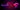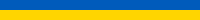# TensorFlow on Spark 2.3: The Best of Both WorldsMay 29, 2018

Categories
Data Science
DataWorks Summit 2018
Tags
Mesos
C++
CPU
GPU
Tuning
Spark
YARN
JavaScript
Keras
Kubernetes
Machine Learning
Python
TensorFlow
Do you like our work......we hire!

Never miss our publications about Open Source, big data and distributed systems, low frequency of one email every two months.

The integration of TensorFlow With Spark has a lot of potential and creates new opportunities.

This article is based on a conference seen at the DataWorks Summit 2018 in Berlin. It was about the new features of the 2.3 release of Apache Spark, an open source framework for Big Data computation on clusters. And one of the aspects the talker insisted on was the integration of Tensorflow in Spark and the benefits of combining them.

Since I am mostly interested in Deep Learning, I was wondering why should I use this software combo to train and use my models. And it turns out we can use both power for a multitude of usecases. So first, let’s try to use a deep learning model for computer vision using TensorFlow in Apache Spark 2.3.

## Tensorflow

TensorFlow is Google’s open source software library dedicated to high performance numerical computation. Taking advantages of GPUs, TPUs and CPUs power and usable on servers, clusters and even mobiles, it is mainly used for Machine Learning and especially Deep Learning.

It provides support for C++, Python and recently for Javascript with TensorFlow.js. The library is now in its 1.8th version and comes with an official high level wraper called Keras.

Tensorflow uses Cuda and CudNN from Nvidia to allow communication with the GPUs. Contrary to CPUs, GPUs are designed to perform parallel tasks and Matrix Operations, which are heavily present in Machine Learning, Deep Learning and Data computation, can profit of parallel computing.

Since matrix multiplication is one of the most heavy computation for matrices let’s have a look at some benchmarks and then try to implement it with and without Tensorflow.

### Matrix Multiplication

As we can see, past a certain dimension, matrix multiplication computation time explodes with CPUs whereas for GPU it stays very low.

### Without Tensorflow

``````import numpy as np

m1 = np.random.normal(0, 0.2, size=(800, 800))
m2 = np.random.normal(0, 0.2, size=(800, 800))

# Evaluate
result = m1 @ m2

# Show results
print(result)``````

### With Tensorflow

``````import tensorflow as tf
import numpy as np

m1 = np.random.normal(0, 0.2, size=(800, 800))
m2 = np.random.normal(0, 0.2, size=(800, 800))

# Define Placeholders and Operation Graph
M1 = tf.placeholder(tf.float32, shape=(800, 800), name='M1') # M1: matrix 28x28
M2 = tf.placeholder(tf.float32, shape=(800, 800), name='M2') # M2: matrix 28x28
output = tf.matmul(M1, M2)

# Evaluate graph
with tf.Session() as sess:
result = sess.run(output, feed_dict = {M1: m1, M2: m2})

# Show results
print(result)``````

## Apache Spark

Apache Spark is an efficient scalable open source framework dedicated to Big Data. It is good at running heavy computations on clusters and distributing them. It is simple of usage and fast. Simpler than Hadoop, Spark allows you to develop in Java, Scala, Python and R. It is 100 times faster than Hadoop MapReduce.

Spark is also general. It can be used as a SQL query engine and can handle streaming thanks to Spark Streaming. Moreover, it comes with a Machine Learning Library called MLLib.

The framework can be executed on Hadoop 2 clusters with YARN, Mesos, Kubernetes or in standalone and allows access to multiple data sources such as HDFS, Cassandra, HBase and Amazon S3.

The new release of Spark: Apache Spark 2.3, comes with multiple features.

### Retrieve Images from a file system

Here’s a sample code to retrieve images from filesystem with PySpark

``````from pyspark.ml.image import ImageSchema

# Define images path
IMAGES_PATH = "datasets/image_classifier/test/"

# Create a spark DataFrame for images

# Show DataFrame
images_df.show()``````

## TensorFlow x Apache Spark 2.3: TensorFrame

With the 2.3 release, Tensorflow is supported by Apache Spark. Thus we can combine the power of DataFrames, Transformers and Estimators to TensorFlow and Keras.

``````from keras.applications import InceptionV3

# Load inception v3 (Best Image Classifier)
model = InceptionV3(weights="imagenet")

# Save the model
model.save('/tmp/model-full.h5')
from keras.applications.inception_v3 import preprocess_input
import numpy as np
import os
from pyspark.sql.types import StringType
from sparkdl import KerasImageFileTransformer

# Parameters
SIZE = (299, 299)  # Size accepted by Inception model
IMAGES_PATH = 'datasets/image_classifier/test/'  # Images Path
MODEL = '/tmp/model-full-tmp.h5'  # Model Path

# Image Preprocessing
def preprocess_keras_inceptionV3(uri):
image = np.expand_dims(image, axis=0)
return preprocess_input(image)

# Define Spark Transformer
transformer = KerasImageFileTransformer(inputCol="uri", outputCol="predictions",
modelFile=MODEL,
outputMode="vector")

# Get Input
files = [os.path.abspath(os.path.join(dirpath, f)) for f in os.listdir(IMAGES_PATH) if f.endswith('.jpg')]
uri_df = sqlContext.createDataFrame(files, StringType()).toDF("uri")

# Get Output
labels_df = transformer.transform(uri_df)

# Show Output
labels_df.show()``````

As you can see it is pretty straightforward. You just have to turn your model into a transformer or an estimator.

## Use cases

Hyper parameter selection can be time consuming. Moreover, one small change can have huge consequences. As it’s nearly impossible to test out every combination, it’s at least possible to perform some fine tuning by giving each node of a Spark cluster a different set , run them synchronously, then cross validate to pick the best one.

Tensorflow in Spark 2.3 can also be usefull for model deployment and scalability. Thanks to Spark, we can broadcast a pretrained model to each node and distribute the predictions over all the nodes. Regarding scaling, Spark allows new nodes to be added to the cluster if needed.

## Conclusion

To conclude, this new Spark release offers the possibility to apply the benefits of distributed computation to the Deep Learning/Machine Learning field.